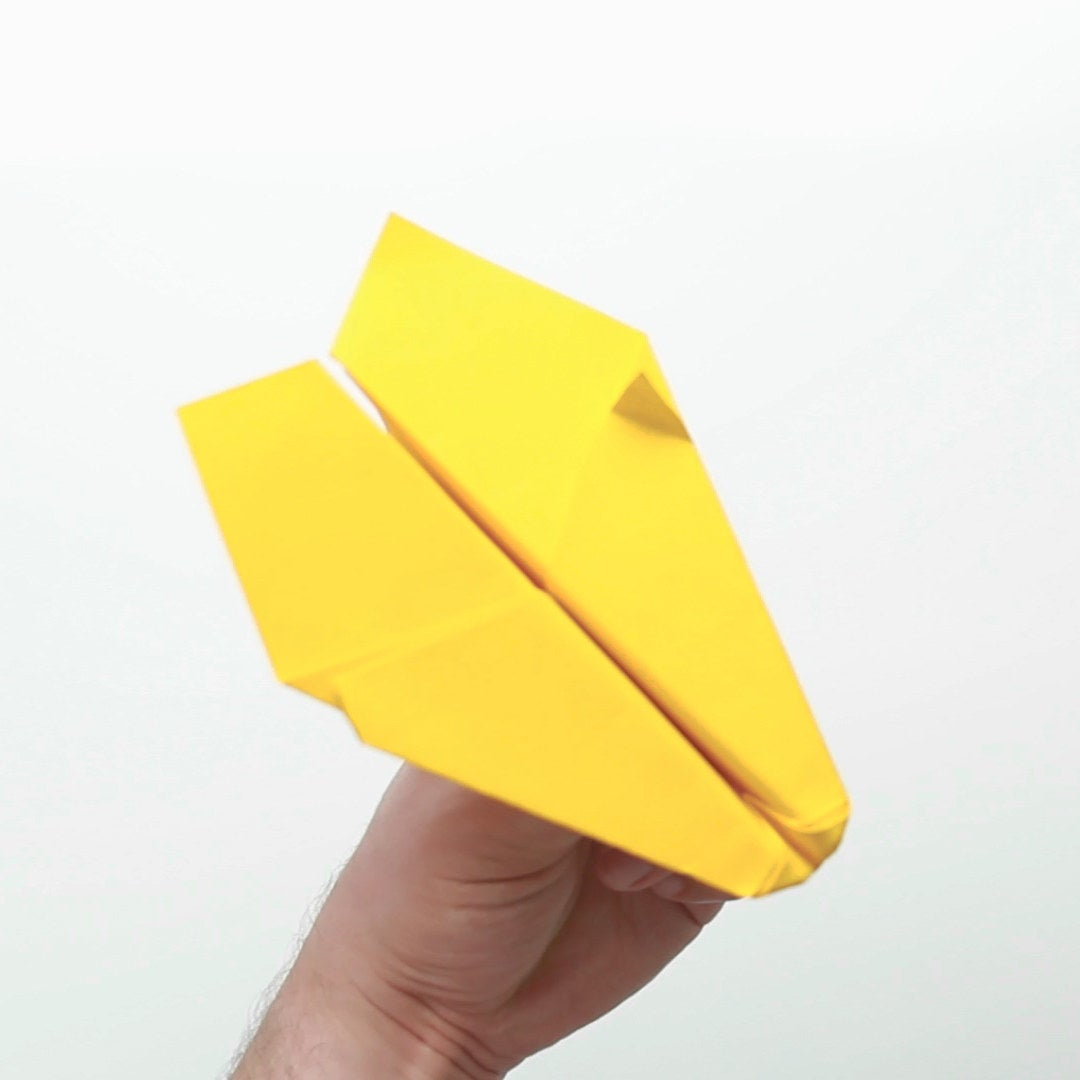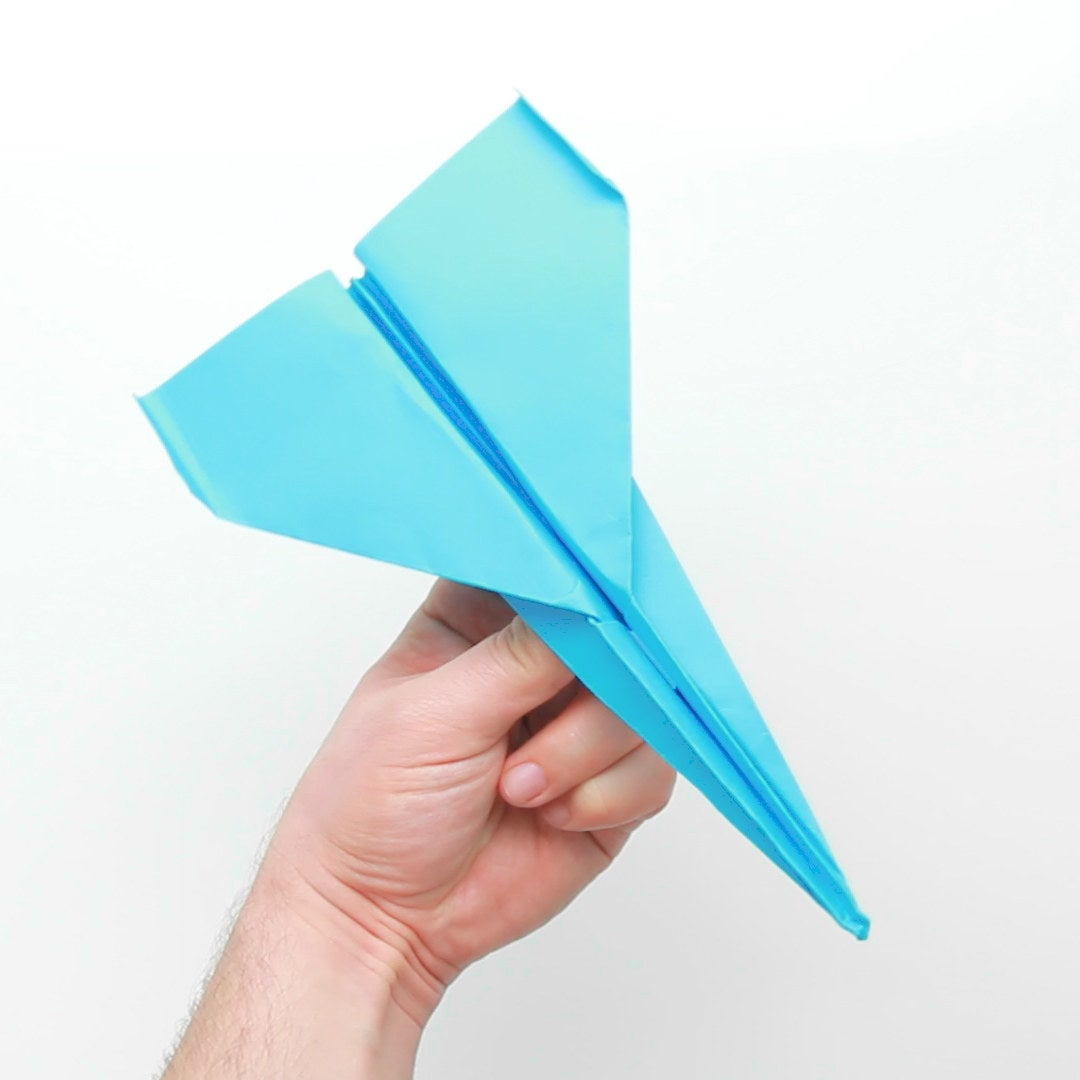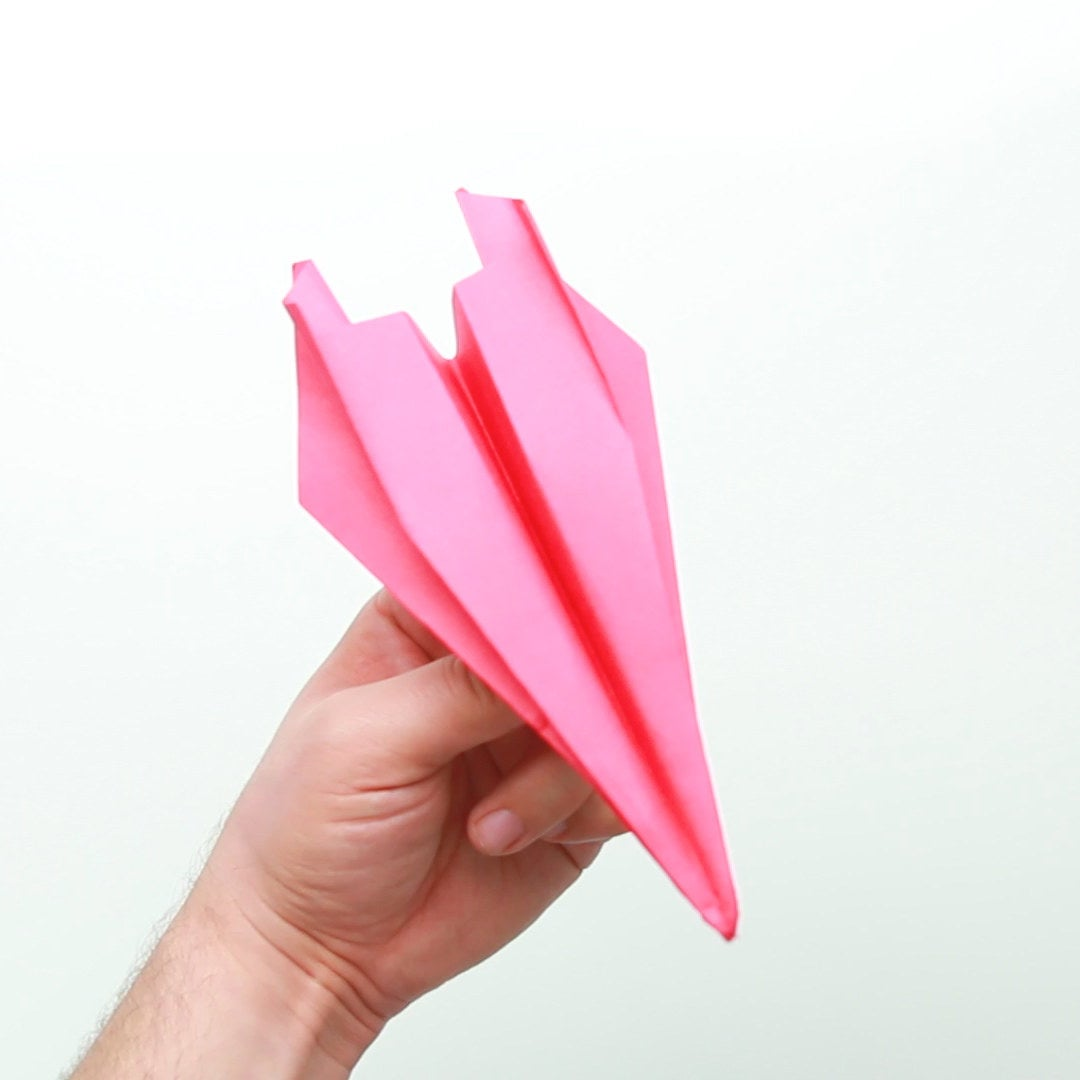Fly, fly away.

View Video ›

# Sun Cruiser1. Fold a right triangle from the left side of the colored paper.
2. Unfold the paper back to the original position
3. Fold a right triangle from the right side
4. Unfold the paper and creases in the form of an “X” should be appear.
5. Fold the left and right triangle from the “X” crease inward.
6. The fold should now be a triangle with a rectangle underneath it.
7. Use the tip of your thumb to fold the highest point inward.
8. Now fold the paper in half.
9. As the folded paper is on its side, fold the paper in half, outward. Matching the other edges.
10. Use your thumb to fold the smaller triangle, outward
11. Now flip the paper over to the other side and repeat steps 9 and 10.
12. Unfold the two folded sides to form the wings of the plane.
13. Straighten the wings so they are even.

BuzzFeed

# Cloud Seeker1. Fold the colored paper in half using the vertical plane.
2. Fold the top-right corner inward, forming a right triangle.
3. Fold the top-left corner inward, forming a right triangle.
4. Fold the paper outward so that there is a small gap from the tip of the triangle and the bottom.
5. Use the bottom gap space to fold the paper so the triangle is underneath the gap and top layer.
6. Using the left half of the paper, fold the lower portion inward and the upper layer of paper outward so the paper has a crest at the top.
7. Now use the right half of the paper and fold the lower portion inward and the upper layer outward so the paper matches the opposing side.
8. Now the paper should have four layers from bottom to top.
9. Fold the left side of the top layer inward in half.
10. Fold the right side of the top layer inward in half.
11. Fold all the layers outward in half.
12. Fold the tips of each wing outward.
13. Fold the bottom right corner inward to form a right triangle.
14. Unfold the halves of layers to reveal the paper airplane.
15. Use a finger to prop up the right triangle fold located in the middle of the wings. The fold should now look like a fin.
16. Straighten the folds and release the paper airplane.

BuzzFeed

# Flame Rocket1. Fold the paper in half using the vertical plane.
2. Fold the upper right corner inward, forming a right triangle. Unfold the the paper.
3. Now use the upper left corner and fold it inward, forming a right triangle. Unfold the paper. The paper should now have creases that intersect from the folds.
4. Fold the left and right triangles inside of the creases inward.
5. Turn over the left folded triangle.
6. Fold the left side toward the center of the folds.
7. Flip the 2 triangles over the folded side.
8. Now fold the right side toward the center to mirror the opposing side.
9. Flip the triangles over to the right side.
10. Fold the wing outward towards the edge.
11. Flip 1 triangle to the left, over the folded wing.
12. Flip the entire paper over.
13. Fold the right side to match the left side.
14. Flip the fold over to the right side.
15. Fold the outer layer outward to match the opposite side.
16. Flip the entire paper back over.
17. Fold the left side of the top triangle towards the center.
19. Fold the right side of the top triangle towards the center.
20. Tuck the center edges inside of the bottom layer.
21. Fold the layers in half outward.
22. Fold the right edge toward the center.
23. Flip over the layers.
24. Fold the left edge in half.
25. Unfold the wings.
26. Fold the tips of the wings vertically.
27. Straighten the folds and release the paper airplane.

BuzzFeed

View Entire List ›

Source: https://www.buzzfeed.com/diy.xml

This site uses Akismet to reduce spam. Learn how your comment data is processed.